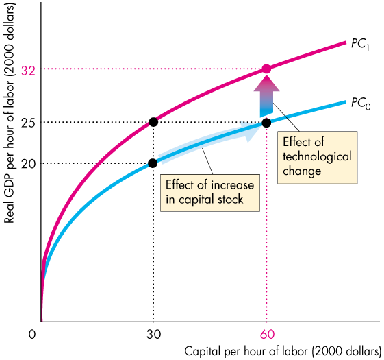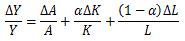### Why should I choose AnalystNotes?

Simply put: AnalystNotes offers the best value and the best product available to help you pass your exams.

##### Subject 3. Production Function and Growth Accounting
Production Function

Y = A F(K,L)

The quantity of real GDP supplied, Y, depends on the quantity of labor, L, the quantity of capital, K, and A which is referred to as total factor productivity (TFP).

• More inputs mean more output. That is, the marginal product of labor and the marginal production of capital are both positive.
• The higher of the level of technology, the more output is produced for a given level of inputs. Both the function F() and A reflect technology.

The Cobb-Douglas production function is widely used to represent the relationship of output and inputs of capital and labor.

F(K,L) = Kα L1-α
where 0 < α < 1.

Two important properties:

• Constant returns to scale.
• Diminishing marginal productivity.

The productivity curve is the relationship between real GDP per hour of labor and the amount of capital per hour of labor, with technology held constant.Productivity can grow for two reasons.

• Capital Deepening: An increase in the capital-to-labor ratio brings a movement along productivity curve.

The shape of the productivity curve reflects the law of diminishing returns: As the quantity of one input increases with the quantities of all other inputs remaining the same, output increases but ever smaller increments. As capital-to-labor ratio rises, output rises (the marginal product of capital is positive), but output rises less at high levels of capital than at low levels. This is the key explanation of why the economy reaches a steady state rather than growing endlessly.

• Technology Progress: Technological change shifts the productivity curve.

An advance in technology shifts the productivity curve from PC0 to PC1.

Growth Accounting

Growth accounting explains what part of growth in total output is due to growth in different factors of production (i.e., capital, labor, technology). It is also known as "sources of growth analysis".The equation is essentially the same as the production function written in the form of growth rates.

User Comment
Oksanata "alpha" determines the shares of output paid by companies to Capital and Labor.
CFAJ thank you santa :P